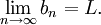# Squeeze Theorem for Sequences

Previous: Example Relating Sequences of Absolute Values

Next: Squeeze Theorem Example

The Squeeze Theorem for functions can also be adapted for infinite sequences.

Theorem: Squeeze Theorem for Infinite Sequences
SupposeforandthenThis theorem allows us to evaluate limits that are hard to evaluate, by establishing a relationship to other limits that we can easily evaluate. Let's see this in an example.

Previous: Example Relating Sequences of Absolute Values

Next: Squeeze Theorem Example